# Percentage - Quantitative Aptitude (MCQ) questions

Dear Readers, Welcome to Quantitative Aptitude Percentage questions and answers with explanation. These Percentage solved examples with shortcuts and tricks will help you learn and practice for your Placement Test and competitive exams like Bank PO, IBPS PO, SBI PO, RRB PO, RBI Assistant, LIC,SSC, MBA - MAT, XAT, CAT, NMAT, UPSC, NET etc.

After practicing these tricky Percentage multiple choice questions, you will be exam ready to deal with any objective type questions.

1)   A’s salary is 50% more than B’s. How much percent is B’s salary less than A’s?
- Published on 11 Apr 17

a. 33(1/4)%
b. 33(1/3)%
c. 33(1/2)%
d. 33%
 Answer  Explanation ANSWER: 33(1/3)% Explanation: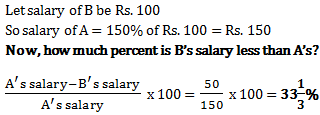2)   The price of cooking oil has increased by 25%. By what percent should a family reduce the consumption of cooking oil so as not to increase the expenditure in this account?
- Published on 11 Apr 17

a. 20%
b. 25%
c. 18%
d. 16%
 Answer  Explanation ANSWER: 20% Explanation: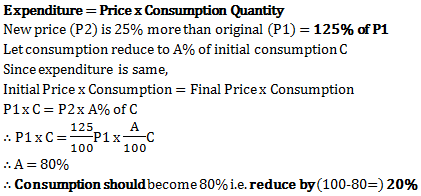3)   Ramesh’s salary was reduced by 10% and then the reduced salary was increased by 10%. What was his ultimate loss?
- Published on 11 Apr 17

a. 0%
b. 10%
c. 1%
d. 5%
 Answer  Explanation ANSWER: 1% Explanation: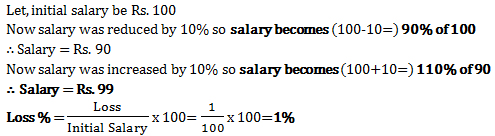4)   In a country 55% population is female. 80% of the male population is literate. How much of females are literate if total literacy is 58%?
- Published on 11 Apr 17

a. 45%
b. 55%
c. 40%
d. 22%
 Answer  Explanation ANSWER: 40% Explanation: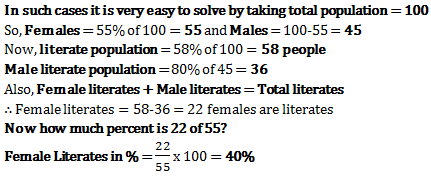5)   Two numbers are less than a third number by 30% and 37% respectively. How much percent is the second number less than the first?
- Published on 05 Apr 17

a. 7%
b. 10%
c. 4%
d. 3%
 Answer  Explanation ANSWER: 10% Explanation: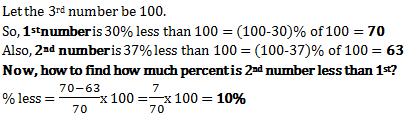6)   40% of greater number is equal to 60% of the smaller. If their sum is 150, then the greater number is
- Published on 05 Apr 17

a. 70
b. 80
c. 90
d. 60
 Answer  Explanation ANSWER: 90 Explanation: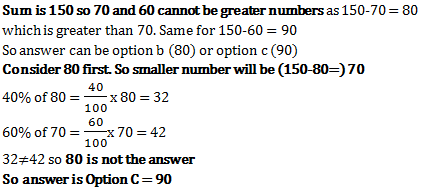7)   If X% of Y is 100 and Y% of Z is 200, find a relation between X and Z.
- Published on 05 Apr 17

a. Z = X/2
b. Z = 2X
c. X = Z/4
d. Z = 4X
 Answer  Explanation ANSWER: Z = 2X Explanation: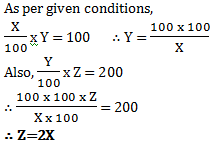8)   If 20% of an electricity bill is deducted, then Rs. 100 is still to be paid. How much was the original bill?
- Published on 05 Apr 17

a. Rs. 110
b. Rs. 115
c. Rs. 120
d. Rs. 125
 Answer  Explanation ANSWER: Rs. 125 Explanation: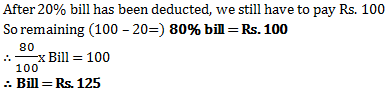9)   5% of 5% of Rs. 100 is
- Published on 05 Apr 17

a. Rs. 0.25
b. Rs. 0.50
c. Rs. 10
d. Rs. 25
 Answer  Explanation ANSWER: Rs. 0.25 Explanation: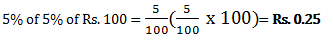10)   A town has population of 50,000 in 1988. In one year i.e. by 1989 it increased by 25%. Next year i.e. in 1990, it decreased by 30%. The next year in 1991 there was an increase of 40%. What is the population at end of 1991?
- Published on 17 Mar 17

a. 60250
b. 62250
c. 66550
d. 61250
 Answer  Explanation ANSWER: 61250 Explanation: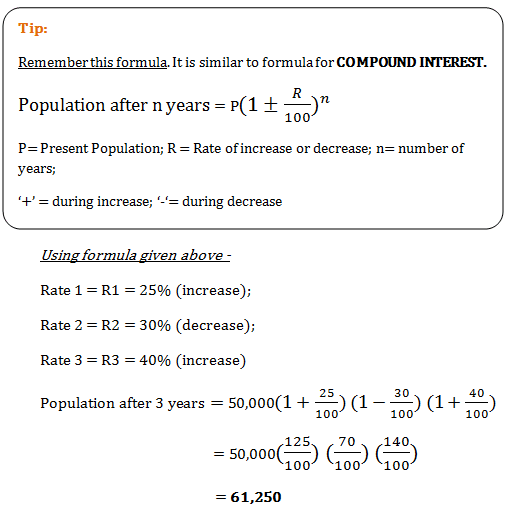1 2 3 4 5